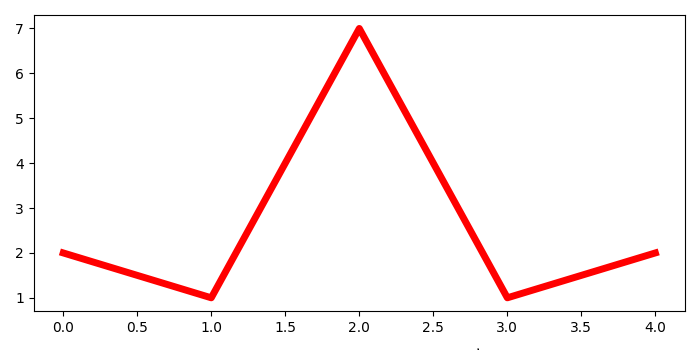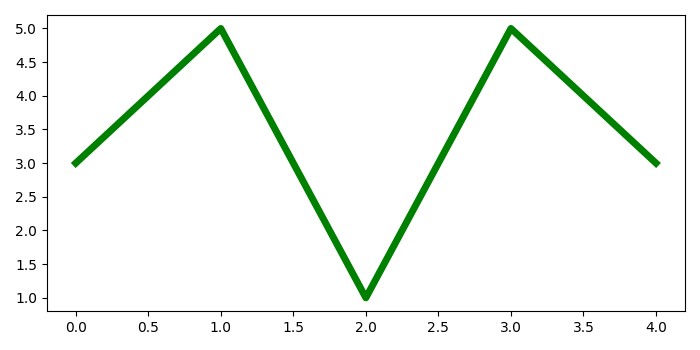# Saving multiple figures to one PDF file in matplotlib

To save multiple figures in one PDF file at once, we can take follwong steps

## Steps

• Set the figure size and adjust the padding between and around the subplots.

• Create a new figure (fig1) or activate and existing figure using figure() method.

• Plot the first line using plot() method.

• Create another figure (fig2) or activate and existing figure using figure() method.

• Plot the second line using plot() method.

• Initialize a variable, filename, to make a pdf file.

• Create a user-defined function save_multi_image() to save multiple images in a PDF file.

• Call the save_multi_image() function with filename.

• Create a new PdfPages object.

• Get the number of open figures.

• Iterate the opened figures and save them into the file.

• Close the created PDF object.

## Example

from matplotlib import pyplot as plt
from matplotlib.backends.backend_pdf import PdfPages

plt.rcParams["figure.figsize"] = [7.00, 3.50]
plt.rcParams["figure.autolayout"] = True

fig1 = plt.figure()
plt.plot([2, 1, 7, 1, 2], color='red', lw=5)

fig2 = plt.figure()
plt.plot([3, 5, 1, 5, 3], color='green', lw=5)

def save_multi_image(filename):
pp = PdfPages(filename)
fig_nums = plt.get_fignums()
figs = [plt.figure(n) for n in fig_nums]
for fig in figs:
fig.savefig(pp, format='pdf')
pp.close()

filename = "multi.pdf"
save_multi_image(filename)


## OutputUpon execution, it will create a PDF "multi.pdf" in the Project Directory and save the following two images in that file.